## 2.5.3 Planning as Satisfiability

Another interesting approach is to convert the planning problem into an enormous Boolean satisfiability problem. This means that the planning problem of Formulation 2.4 can be solved by determining whether some assignment of variables is possible for a Boolean expression that leads to a TRUE value. Generic methods for determining satisfiability can be directly applied to the Boolean expression that encodes the planning problem. The Davis-Putnam procedure is one of the most widely known algorithms for satisfiability. It performs a depth-first search by iteratively trying assignments for variables and backtracking when assignments fail. During the search, large parts of the expression can be eliminated due to the current assignments. The algorithm is complete and reasonably efficient. Its use in solving planning problems is surveyed in . In practice, stochastic local search methods provide a reasonable alternative to the Davis-Putnam procedure .

Suppose a planning problem has been given in terms of Formulation 2.4. All literals and operators will be tagged with a stage index. For example, a literal that appears in two different stages will be considered distinct. This kind of tagging is similar to situation calculus ; however, in that case, variables are allowed for the tags. To obtain a finite, Boolean expression the total number of stages must be declared. Let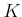denote the number of stages at which operators can be applied. As usual, the fist stage is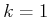and the final stage is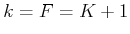. Setting a stage limit is a significant drawback of the approach because this is usually not known before the problem is solved. A planning algorithm can assume a small value for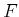and then gradually increase it each time the resulting Boolean expression is not satisfied. If the problem is not solvable, however, this approach iterates forever.

Let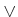denote logical OR, and let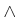denote logical AND. The Boolean expression is written as a conjunction2.5 of many terms, which arise from five different sources:

1. Initial state: A conjunction of all literals in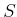is formed, along with the negation of all positive literals not in. These are all tagged with, the initial stage index.
2. Goal state: A conjunction of all literals in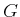, tagged with the final stage index,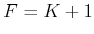.
3. Operator encodings: Each operator must be copied over the stages. For each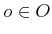, let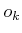denote the operator applied at stage. A conjunction is formed over all operators at all stages. For each, the expression is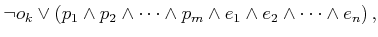(2.33)

in which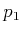,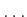,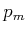are the preconditions of, and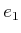,,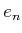are the effects of.
4. Frame axioms: The next part is to encode the implicit assumption that every literal that is not an effect of the applied operator remains unchanged in the next stage. This can alternatively be stated as follows: If a literal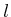becomes negated to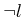, then an operator that includesas an effect must have been executed. (Ifwas already a negative literal, thenis a positive literal.) For each stage and literal, an expression is needed. Suppose that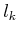and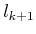are the same literal but are tagged for different stages. The expression is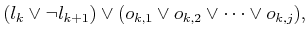(2.34)

in which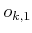,,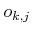are the operators, tagged for stage, that containas an effect. This ensures that if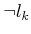appears, followed by, then some operator must have caused the change.
5. Complete exclusion axiom: This indicates that only one operator applies at every stage. For every stage, and any pair of stage-tagged operatorsand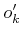, the expression is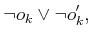(2.35)

which is logically equivalent to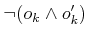(meaning, not both at the same stage'').
It is shown in  that a solution plan exists if and only if the resulting Boolean expression is satisfiable.

The following example illustrates the construction.

Example 2..9 (The Flashlight Problem as a Boolean Expression)   A Boolean expression will be constructed for Example 2.6. Each of the expressions given below is joined into one large expression by connecting them with's.

The expression for the initial state is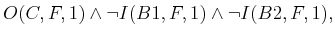(2.36)

which uses the abbreviated names, and the stage tag has been added as an argument to the predicates. The expression for the goal state is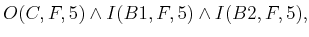(2.37)

which indicates that the goal must be achieved at stage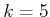. This value was determined because we already know the solution plan from (2.24). The method will also work correctly for a larger value of. The expressions for the operators are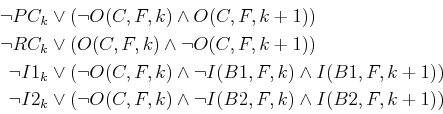(2.38)

for eachfromto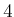.

The frame axioms yield the expressions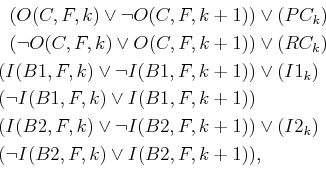(2.39)

for eachfromto. No operators remove batteries from the flashlight. Hence, two of the expressions list no operators.

Finally, the complete exclusion axiom yields the expressions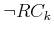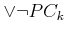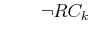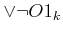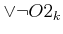(2.40)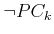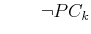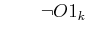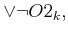for eachfromto. The full problem is encoded by combining all of the given expressions into an enormous conjunction. The expression is satisfied by assigning TRUE values to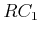,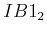,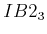, and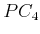. An alternative solution is,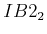,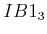, and. The stage index tags indicate the order that the actions are applied in the recovered plan.Steven M LaValle 2020-08-14